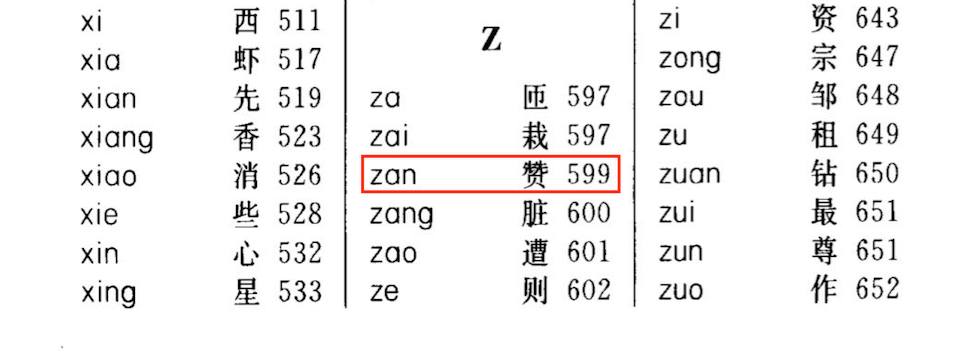01.哈希表知识

# 哈希表知识 #

## 1. 哈希表简介 #

• 向哈希表中插入一个关键字：哈希函数决定该关键字的对应值应该存放到表中的哪个区块，并将对应值存放到该区块中。
• 在哈希表中搜索一个关键字：使用相同的哈希函数从哈希表中查找对应的区块，并在特定的区块搜索该关键字对应的值。• 插入：通过哈希函数解析关键字，并将对应值存放到该区块中。
• 比如：0138 通过哈希函数 Hash(key) = 0138 // 100 = 0，得出应将 0138 分配到0 所在的区块中。
• 查找：通过哈希函数解析关键字，并在特定的区块搜索该关键字对应的值。
• 比如：查找 2321，通过哈希函数，得出 2321 应该在 2 所对应的区块中。然后我们从 2 对应的区块中继续搜索，并在 2 对应的区块中成功找到了 2321
• 比如：查找 3214，通过哈希函数，得出 3214 应该在 3 所对应的区块中。然后我们从 3 对应的区块中继续搜索，但并没有找到对应值，则说明 3214 不在哈希表中。• 存放所有拼音和对应地址的表可以看做是 「哈希表」
• 赞 字的拼音索引 zan 可以看做是哈希表中的 「关键字 key
• 根据拼音索引 zan 可以确定字对应页码的过程可以看做是哈希表中的 「哈希函数 Hash(key)
• 所查找到的对应页码 599 可以看做是哈希表中的 「哈希地址 value

## 2. 哈希函数 #

• 哈希函数应该易于计算，并且尽量使计算出来的索引值均匀分布。
• 哈希函数计算得到的哈希值是一个固定长度的输出值。
• 如果 Hash(key1) 不等于 Hash(key2)，那么 key1key2 一定不相等。
• 如果 Hash(key1) 等于 Hash(key2)，那么 key1key2 可能相等，也可能不相等（会发生哈希碰撞）。

### 2.1 直接定址法 #

• 直接定址法：取关键字或者关键字的某个线性函数值为哈希地址。即：Hash(key) = key 或者 Hash(key) = a * key + b，其中 ab 为常数。

### 2.2 除留余数法 #

• 除留余数法：假设哈希表的表长为 m，取一个不大于 m 但接近或等于 m 的质数 p，利用取模运算，将关键字转换为哈希地址。即：Hash(key) = key % p，其中 p 为不大于 m 的质数。

### 2.3 平方取中法 #

• 平方取中法：先通过求关键字平方值的方式扩大相近数之间的差别，然后根据表长度取关键字平方值的中间几位数为哈希地址。
• 比如：Hash(key) = (key * key) // 100 % 1000，先计算平方，去除末尾的 2 位数，再取中间 3 位数作为哈希地址。

### 2.4 基数转换法 #

• 基数转换法：将关键字看成另一种进制的数再转换成原来进制的数，然后选其中几位作为哈希地址。
• 比如，将关键字看做是 13 进制的数，再将其转变为 10 进制的数，将其作为哈希地址。

343246 为例，哈希地址计算方式如下：

$343246_{13} = 3 \times 13^5 + 4 \times 13^4 + 3 \times 13^3 + 2 \times 13^2 + 4 \times 13^1 + 6 \times 13^0 = 1235110_{10}$

## 3. 哈希冲突 #

### 3.1 开放地址法 #

• H(i) 是在处理冲突中得到的地址序列。即在第 1 次冲突（i = 1）时经过处理得到一个新地址 H(1)，如果在 H(1) 处仍然发生冲突（i = 2）时经过处理时得到另一个新地址 H(2) …… 如此下去，直到求得的 H(n) 不再发生冲突。
• Hash(key) 是哈希函数，m 是哈希表表长，对哈希表长取余的目的是为了使得到的下一个地址一定落在哈希表中。
• F(i) 是冲突解决方法，取法可以有以下几种：
• 线性探测法：$F(i) = 1, 2, 3, …, m - 1$。
• 二次探测法：$F(i) = 1^2, -1^2, 2^2, -2^2, …, \pm n^2(n \le m / 2)$。
• 伪随机数序列：$F(i) = 伪随机数序列$。

• 使用线性探测法：得到下一个地址 H(1) = (5 + 1) % 11 = 6，仍然冲突；继续求出 H(2) = (5 + 2) % 11 = 7，仍然冲突；继续求出 H(3) = (5 + 3) % 11 = 88 对应的地址为空，处理冲突过程结束，记录填入哈希表中序号为 8 的位置。
• 使用二次探测法：得到下一个地址 H(1) = (5 + 1*1) % 11 = 6，仍然冲突；继续求出 H(2) = (5 - 1*1) % 11 = 44 对应的地址为空，处理冲突过程结束，记录填入哈希表中序号为 4 的位置。
• 使用伪随机数序列：假设伪随机数为 9，则得到下一个地址 H(1) = (9 + 5) % 11 = 33 对应的地址为空，处理冲突过程结束，记录填入哈希表中序号为 3 的位置。### 3.2 链地址法 #

• 这样在插入关键字的时候，我们只需要通过哈希函数 Hash(key) 计算出对应的哈希地址 i，然后将其以链表节点的形式插入到以 T[i] 为头节点的单链表中。在链表中插入位置可以在表头或表尾，也可以在中间。如果每次插入位置为表头，则插入操作的时间复杂度为 $O(1)$。

• 而在在查询关键字的时候，我们只需要通过哈希函数 Hash(key) 计算出对应的哈希地址 i，然后将对应位置上的链表整个扫描一遍，比较链表中每个链节点的键值与查询的键值是否一致。查询操作的时间复杂度跟链表的长度 k 成正比，也就是 $O(k)$。对于哈希地址比较均匀的哈希函数来说，理论上讲，k = n // m，其中 n 为关键字的个数，m 为哈希表的表长。## 4. 哈希表总结 #

• 哈希表（Hash Table）：通过键 key 和一个映射函数 Hash(key) 计算出对应的值 value，把关键码值映射到表中一个位置来访问记录，以加快查找的速度。
• 哈希函数（Hash Function）：将哈希表中元素的关键键值映射为元素存储位置的函数。
• 哈希冲突（Hash Collision）：不同的关键字通过同一个哈希函数可能得到同一哈希地址。

• 常用的哈希函数方法有：直接定址法、除留余数法、平方取中法、基数转换法、数字分析法、折叠法、随机数法、乘积法、点积法等。
• 常用的哈希冲突的解决方法有两种：开放地址法和链地址法。